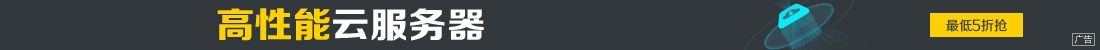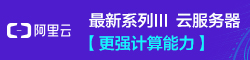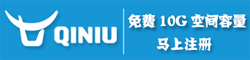# python数据类型_字符串常用操作(详解)

••1.python字符串

```name = 'hello'
name1 = "hello bei jing "
name2 = '''hello shang hai haha'''```

python中的字符串一旦声明，是不能进行更改的，如下：

```#字符串为不可变变量，即不能通过对某一位置重新赋值改变内容

name = 'hello'
name = 'k' #通过下标来修改字符串的值，报错信息：TypeError: 'str' object does not support item assignment
```

python 字符串常用的内置方法，对字符串进行操作，如下：

in方法：

``` #in方法:判断内容是否存在字符串中
name = 'hello bei jing zao an '
print('hello' in name)  #判断hello 是否存在name里,执行结果为True
print('shang hai ' in name) #判断 shanghai 是否存在name里,执行结果为False```

not方法：

```name = 'hello bei jing zao an ' #定义字符串
#not方法：返回表达式结果的'相反值'。如果表达式结果为真，则返回False
print('hello' not in name) #判断hello 不存在name里，执行结果为False
print('abc' not in name) #判断abc 不存在name里，执行结果为True```

is方法：

```#is 判断的是 内存地址是否相同
a = 'xiaoming'
b = 'xiaoming'
print(a is b ) #a和b的值相同，内存里存放时，指向的内存地址相同，指向结果为True
print(id(a))
print(id(b)) #可以通过id(变量名)这个方法，查看变量的内存地址```

```names = 'hello bei jing one day'
print(names[0:10]) # 1.可以通过下标进行取值，切片，顾头不含尾，执行结果是：hello bei
for name in names:
print(name)   #2.通过for循环取值，循环的是循环对象(names)里面的每一个元素
for k in range(len(names)):
print(names[k]) #3.循环names的长度，进行取值，k的值是数字，相当于字符串的下标```

```name = 'hello world is world'
print(name.endswith('d')) #判断是否以u结尾,执行结果为布尔值，工作中的例子：可以判断图片是否以jpg结尾
print('ab123'.isalnum())  #判断输入的字符串是否包含数字和字母，判断密码是否包含数字和字母时，可以使用，返回结果为布尔值
print('abcdA'.isalpha())  #判断输入的字符串是否是英文字母，返回结果为布尔值```

```name = ' abcdERF123'
print('123'.isdigit()) #判断输入的字符串是否为数字，返回结果为布尔值```

```#去除空格
print('  ab fs'.lstrip())   #默认去掉字符串左边的空格和换行,执行结果：ab fs
print('hello  '.rstrip())   #默认去掉字符串右边的空格和换行，执行结果：hello
print('\nmysql abcd'.strip()) #默认去掉两边的空格和换行,执行结果：mysql abcd，中间的空格不可去除
print('mysqlmy'.strip('m'))  #去除指定的字符串，例如：去除两边的m元素,执行结果：ysqlm   y```

```#join是用来通过 某个字符串 拼接 一个可迭代对象的每个元素--->join(可迭代对象参数类型)
print('*'.join(name))   #将字符串中的每个元素都使用*号连接，执行结果：a*b*c*d*E*R*F*1*2*3，返回一个新的变量值
print('使用*号拼接后的name值：', name) #name字符串的内容未进行更改，执行结果：abcdERF123
nums = ['ybq', 'lhl', 'lsh']
print('，'.join(nums))   #将列表转换为字符串，每个元素之间使用逗号连接，执行结果为：ybq，lhl，lsh
#另一种方法将列表转换为字符串
# nums = ['ybq', 'lhl', 'lsh']
# temp = ''
# for i in nums:
#   temp = temp+i+','   #列表转换为字符串，强制类型转换，执行结果为：ybq，lhl，lsh
# print(temp.strip(','))```

```st = 'mysql is db mysql mysql mysql'
print(st.replace('mysql', 'oracle'))  #将字符串中的mysql替换为oracle
print(st.replace('mysql', 'oracle', 1))  #替换的元素存在较多时，可以输入想替换的次数```

```name = 'hello world is world'
print(name.find('world'))  #查找字符串的索引
print(name.find('world', 3, 10)) #可以指定查找字符串的范围，3，15 是开始、结束的下标值,下标值顾头不顾尾
print(name.find('xxx')) #查找的字符串不存在时，返回结果为-1
print(name.rfind('world')) #查找字符串，从后往前查找，执行结果为：15```

```#切割字符串,返回的类型是list
name1 = 'zcl,py,zyz,ywq' #将字符串切割成list
name1_list = name1.split(',') #按照逗号分割字符串，返回结果为list，name1的值未改变
print(name1_list)   #执行结果为list类型：['zcl', 'py', 'zyz', 'ywq']
print(name1.split()) #按照空格分割字符串，返回结果是list，只有一个元素，执行结果：['zcl,py,zyz,ywq']
print(name1.spilt('\n')) #按照换行符分割字符串```

`print('1+2+3\n1+3+4'.splitlines())  #按照换行符分割,分割的是每一行文件的内容作为list的一个元素，执行结果：['1+2+3', '1+3+4']`

```import string
print(string.ascii_letters + string.digits) #输出所有的大小写字母+（0-9）的数字
print(string.ascii_letters)  #输出大小写的英文字母,执行结果：abcdefghijklmnopqrstuvwxyzABCDEFGHIJKLMNOPQRSTUVWXYZ
print(string.ascii_lowercase) #输出小写英文字母，执行结果：abcdefghijklmnopqrstuvwxyz
print(string.ascii_uppercase) #输出小写英文字母，执行结果：ABCDEFGHIJKLMNOPQRSTUVWXYZ```

```print(name.format(name='byz', age=18)) #格式化字符串显示
print(name.format_map({'name': 'zhangsan', 'age': 18})) #格式化字典```

```name = ' abcdERF123'
print('aa'.islower())  #判断输入的字符串是否为小写字母，返回结果为布尔值
print('ASD'.isupper()) #判断输入的字符串是否为大写字母
print(name.lower())   #将字符串中的大写字母变成小写，执行结果：abcderf123
print(name.upper())   #将字符串中的小写字母变成大写，执行结果：ABCDERF123
print('ABCdef'.swapcase()) #大小写字母反转，执行结果：abcDEF```

```#映射
p = str.maketrans('abcdefg', '1234567') #前面的字符串和后面的字符串进行映射，a-->1，c-->3
print('ccaegg'.translate(p)) #输出结果按照上面的maketrans做映射后的字符串，执行结果为：331577
#反解映射
new_p = str.translate('1234567', 'abcdefg')
print('ccaegg'.translate(new_p)) #输出结果按照上面的maketrans做映射后的字符串，执行结果为：ccaegg```

```name = 'hello world is world'
print(name.capitalize()) #首字母大写，执行结果：Hello world
print(name.center(50, '*')) #长度总共为50，将name字符串的值放在中间，两边补充*号显示```